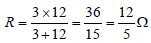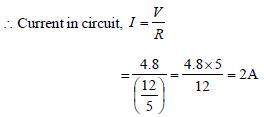Courses

# Test: Current Electricity 3 - From Past 28 Years Questions

## 30 Questions MCQ Test Physics 28 Years Past year papers for NEET/AIPMT Class 12 | Test: Current Electricity 3 - From Past 28 Years Questions

Description
This mock test of Test: Current Electricity 3 - From Past 28 Years Questions for Class 12 helps you for every Class 12 entrance exam. This contains 30 Multiple Choice Questions for Class 12 Test: Current Electricity 3 - From Past 28 Years Questions (mcq) to study with solutions a complete question bank. The solved questions answers in this Test: Current Electricity 3 - From Past 28 Years Questions quiz give you a good mix of easy questions and tough questions. Class 12 students definitely take this Test: Current Electricity 3 - From Past 28 Years Questions exercise for a better result in the exam. You can find other Test: Current Electricity 3 - From Past 28 Years Questions extra questions, long questions & short questions for Class 12 on EduRev as well by searching above.
QUESTION: 1

### The masses of the three wires of copper are inthe ratio of 1 : 3 : 5 and their lengths are in theratio of 5 : 3 : 1. The ratio of their electricalresistance is 

Solution: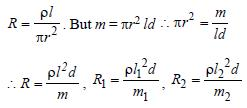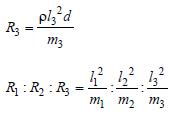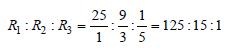QUESTION: 2

### Two batteries of emf 4 V and 8V with internal resistance 1 Ω and 2 Ω are connected in a circuit with a resistance of 9 Ω as shown in figure. The current and potential difference between the points P and Q are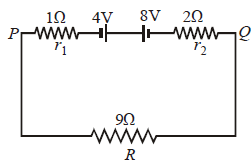Solution: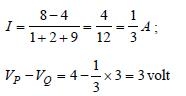QUESTION: 3

### n equal resistors are first connected in seriesand then connected in parallel. What is the ratioof the maximum to the minimum resistance ?

Solution:

In series, Rs= nR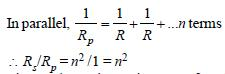QUESTION: 4

Forty electric bulbs are connected in series acrossa 220 V supply. After one bulb is fused theremaining 39 are connected again in seriesacross the same supply. The illumination will be     

Solution:

Since, the voltage is same for the two
combinations, therefore H ∝ 1/R. Hence, the
combination of 39 bulbs will glow more.

QUESTION: 5

A current of 2 A, passing through a conductorproduces 80 J of heat in 10 seconds. Theresistance of the conductor in ohm is 

Solution: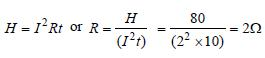QUESTION: 6

Two identical batteries each of e.m.f 2V andinternal resistance 1Ω are available to produceheat in an external resistance by passing a currentthrough it. The maximum power that can bedeveloped across R using these batteries is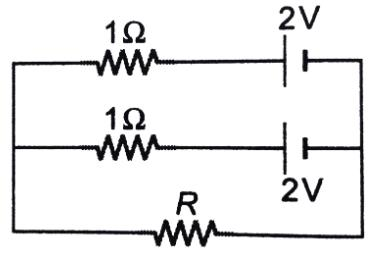Solution:

For maximum current, the two batteries
should be connected in series. The current
will be maximum when external resistance is
equal to the total internal resistance of cells
i.e. 2Ω. Hence power devloped across the
resistacne R will be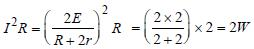QUESTION: 7

You are given several identical resistances eachof value R = 10Ω and each capable of carrying amaximum current of one ampere. It is required tomake a suitable combination of these resistancesof 5Ω which can carry a current of 4 ampere. Theminimum number of resistances of the type Rthat will be required for this job is 

Solution:

To carry a current of 4 ampere, we need four
paths, each carrying a current of one ampere.

Let r be the resistance of each path. These
are connected in parallel. Hence, their
equivalent resistance will be r/4. According
to the given problem r/4 = 5 or r = 20Ω
For this propose two resistances should be
connected. There are four such
combinations. Hence, the total number of
resistance = 4 × 2 = 8.

QUESTION: 8

In the network shown in the Fig, each resistance is 1Ω. The effective resistance between A and B  is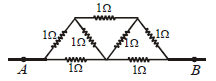Solution:

At A current is distributed and at B currents
are collected. Between A and B, the
distribution is symmetrical. It has been
shown in the figure. It appears that current
in AO and OB remains same. At O, current i4
returns back without any change. If we
detach O from AB there will not be any
change in distribution.
Now, CO & OD will be in series hence its
total resistance = 2Ω
It is in parallel with CD, so, equivalent
resistance =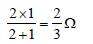This equivalent resistance is in series with
AC & DB, so, total resistance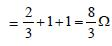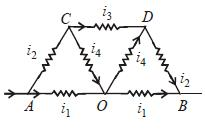Now 8/3 Ω is parallel to AB, that is, 2Ω so
total resistance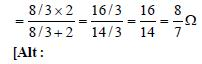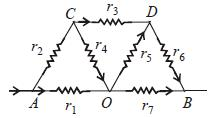Between C & D, the equivalent resistance is
given by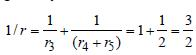Equivalent resistance along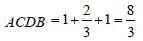∴ Effective resistance between A and B is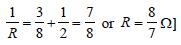QUESTION: 9

Faraday’s laws are consequence of conservationof 

Solution:

Faraday’s laws are based on the conversion
of electrical energy into mechanical energy;
which is in accordance with the law of
conservation of energy

QUESTION: 10

The velocity of charge carriers of current (about1 amp) in a metal under normal conditions is ofthe order of 

Solution: The velocity of charge carriers of current 1 ampere in ametal under normal conditions is of the order of a fraction ofmm/sec
QUESTION: 11

Direct current is passed through a coppersulphate solution using platinum electrodes. Theelements liberated at the electrodes are 

Solution:

In the electrolysis of CuSO4, oxygen is
liberated at anode and copper is deposited
at cathode.

QUESTION: 12

Three resistances each of 4 Ω are connected toform a triangle. The resistance between any twoterminals is 

Solution:

The two resistances are connected in series
and the resultant is connected in parallel with
the third resistance.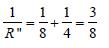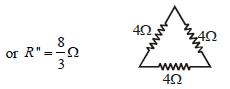QUESTION: 13

Current through 3 Ω resistor is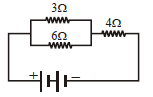0.8 amp., then potential drop through 4Ω resistor is           

Solution:

Voltage across 3 Ω resistance = 3 × 0.8 = 2.4V
This voltage is the same across 6 Ω
resistance. Hence current through this
resistance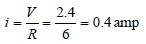Total current in the circuit
= 0.8 + 0.4 = 1.2 amp
Voltage across 4 Ω resistance
= 4 × 1.2 = 4.8 volts

QUESTION: 14

A 4 μF capacitor is charged to 400 volts andthen its plates are joined through a resistance of1k Ω. The heat produced in the resistance is           

Solution:

The energy stored in the capacitor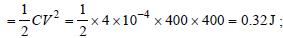This energy will be converted into heat in
the resistor.

QUESTION: 15

In the circuit shown in Fig, the current in 4 Ω resistance is 1.2 A. What is the potential difference between B and C.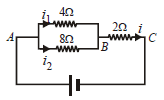Solution:

The potential difference across 4Ω resistance
is given by

V = 4 × i1 = 4 × 1.2 = 4.8 volt

So, the potential across 8Ω resistance is also
4.8 volt.Current in 2Ω resistance i = i1 + i2
∴ i = 1.2 + 0.6 = 1.8 amp
Potential difference across 2Ω resistance
VBC = 1.8 × 2 = 3.6volts

QUESTION: 16

A heating coil is labelled 100 W, 220 V. The coil iscut in half and the two pieces are joined in parallelto the same source. The energy now liberatedper second is 

Solution:

Power of heating coil = 100 W and voltage
(V) = 220 volts. When the heating coil is cut
into two equal parts and these parts are
joined in parallel, then the resistance of the
coil is reduced to one-fourth of the previous
value. Therefore energy liberated per second
becomes 4 times i.e., 4 × 100 = 400 J.

QUESTION: 17

If a wire of resistance R is melted and recasted tohalf of its length, then the new resistance of thewire will be 

Solution:

Initial resistance (R1) = R; Initial length is
1and final length ( 2) = 0.5 . Volume of a
wire = . A. Since the volume of the wire
remains the same after recasting, therefore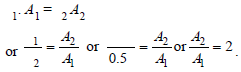We also know that resistance of a wire (R)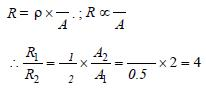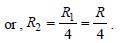[Alt : When wires are drawn from same
volume but with different area of
cross-section, then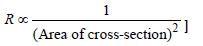QUESTION: 18

Two wires of the same metal have same length,but their cross-sections are in the ratio 3:1. Theyare joined in series. The resistance of thickerwire is 10Ω. The total resistance of thecombination will be 

Solution:

Length of each wire = ; Area of thick wire
(A1) = 3A; Area of thin wire (A2) = A and resistance of thick wire (R1) = 10 Ω

Resistance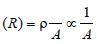(if is constant)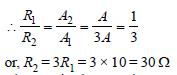The equivalent resistance of these two
resistors in series
= R1 + R, = 30 + 10 = 40Ω.

QUESTION: 19

If the resistance of a conductor is 5 Ω at 50ºCand 7Ω at 100ºC, then the mean temperature coefficient of resistance (of the material) is

Solution:

We know that R(T)=R(0)(1+αT)

where R(T)= resistance at temp T,

R0= resistance at temp T=0oC

and α= temperature coefficient of resistance.

Here, 5=R0(1+α.50)...(1)

and 7=R0(1+α.100)...(2)

(2)/(1)

⇒7/5=(1+100α)/(1+50α)

7+350α=5+500α

150α=2

⇒α=2/150

=0.013/ºC

QUESTION: 20

What will be the equivalent resistance of circuitshown in figure between two points A and D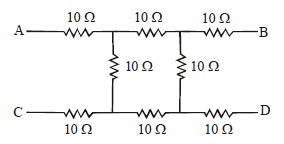Solution: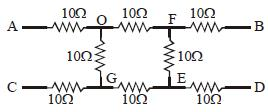Equivalent Circuit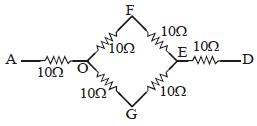Equivalent Resistance of circuit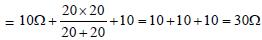QUESTION: 21

If a negligibly small current is passed through awire of length 15 m and of resistance 5Ω havinguniform cross-section of 6 × 10–7 m2 , thencoefficient of resistivity of material, is 

Solution:

Given : Length of wire (l) = 15m
Area (A) = 6 × 10–7 m2
Resistance (R) = 5Ω.
We know that resistance of the wire material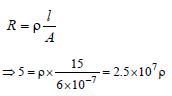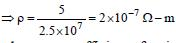QUESTION: 22

Kirchoff’s first law, i.e. ∑i = 0 at a junction, dealswith the conservation of [1992, 1997]

Solution:

We know from the Kirchhoff 's first law that
the algebraic sum of the current meeting at
any junction in the circuit is zero (i.e. ∑i = 0)
or the total charge remains constant.
Therefore, Kirchhoff's first law at a junction
deals with the conservation of charge

QUESTION: 23

A (100 W, 200 V) bulb is connected to a 160Vpower supply. The power consumption would be    

Solution:

Power = 100 W, Voltage = 200 V

Resistance of bulb =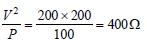When bulb is applied across 160 V,
Current in bulb = 160/400 A

Power consumption =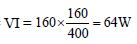QUESTION: 24

There are three copper wires of length and cross sectional area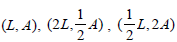.   Inwhich case is the resistance minimum?   

Solution: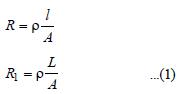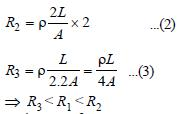QUESTION: 25

The current in the following circuit is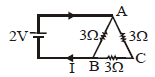Solution:

Resistance of ACB, R' = 3Ω + 3Ω = 6Ω.
For net resistance between A and B; R' = 6Ω
and 3Ω are in parallel.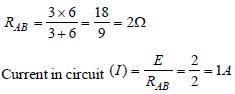QUESTION: 26

Three equal resistors connected across a sourceof e.m.f. together dissipate 10 watt of power.What will be the power dissipated in watts if thesame resistors are connected in parallel acrossthe same source of e.m.f.?      

Solution:

In series, Equivalent resistance = 3R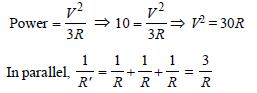∴ Equivalent resistance R' = R/3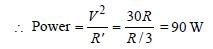QUESTION: 27

If nearly 105 coulombs liberate 1 gm-equivalentof aluminium, then the amount of aluminium(equivalent weight 9), deposited throughelectrolysis in 20 minutes by a current of 50 amp.will be 

Solution:

m = Zit, 9 = Z × 105, Z= 9 × 10–5
Again,
m = Zit = 9 × 10–5 × 50 × 20 × 60 = 5.4 gm

QUESTION: 28

A 5ºC rise in temperature is observed in aconductor by passing a current. When thecurrent is doubled the rise in temperature will beapproximately 

Solution:

Since H ∝ I2 , doubling the current will
produce 4 times heat. Hence, the rise in
temperature will also be 4 times i.e., rise in
temperature = 4 × 5 = 20ºC.

QUESTION: 29

The resistance of a discharge tube is 

Solution:

In discharge tube the current is due to flow
of positive ions and electrons. Moreover,
secondary emission of electrons is also
possible. So V-I curve is non-linear; hence
resistance is non-ohmic.

QUESTION: 30

The current (I) in the given circuit is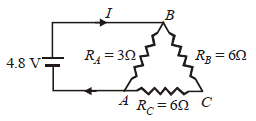Solution:

In circuit, RB and RC are in series, so, Rs = 6 +
6 = 12Ω. This 12 Ω resistance is in parallel
with RA = 3 Ω,
So, equivalent resistance of circuit